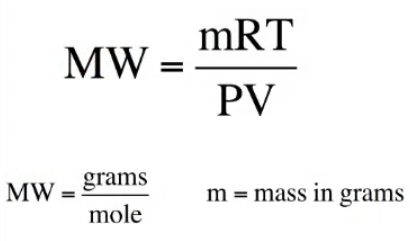# Problem: A piece of solid carbon dioxide, with a mass of 7.8 g, is placed in a 4.0-L otherwise empty container at 27°C. What is the pressure in the container after all the carbon dioxide vaporizes? If 7.8 g solid carbon dioxide were placed in the same container but it already contained air at 740 torr, what would be the partial pressure of carbon dioxide and the total pressure in the container after the carbon dioxide vaporizes?

###### FREE Expert Solution

We would have to use the molar weight derivative of the ideal gas law to solve this problem.So first, calculate the molar weight.

87% (401 ratings)###### Problem Details

A piece of solid carbon dioxide, with a mass of 7.8 g, is placed in a 4.0-L otherwise empty container at 27°C. What is the pressure in the container after all the carbon dioxide vaporizes? If 7.8 g solid carbon dioxide were placed in the same container but it already contained air at 740 torr, what would be the partial pressure of carbon dioxide and the total pressure in the container after the carbon dioxide vaporizes?